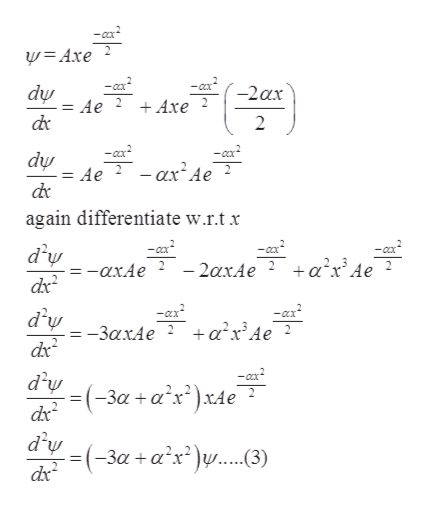# The wavefunction of is Ψ(x) = Axe−αx2/2 for with energy E = 3αℏ2/2m. Find the bounding potential V(x). Looking at the potential’s form, can you write down the two energy levels that are immediately above ??

Question
1 views

The wavefunction of is Ψ(x) = Axe−αx2/2 for with energy E = 3αℏ2/2m
. Find the bounding potential V(x). Looking at the potential’s form, can you write down the two energy levels that are immediately above ??

check_circle

star
star
star
star
star
1 Rating
Step 1

Given:

Step 2

Using wave equation

Step 3

Derivate equation (1) w...help_outlineImage Transcriptionclose-ax y= Axe -CxX -2ax -Ox dự Ae + Axe 2. de -cx? - cx? dự = Ae ? - ax Ae de again differentiate w.r.t x -ca - 2axAe d'y =-AXA. dx - Cox +a'x° Ae ? -ax +a²x³Ae -ax d'y = -3axAe ? dx %3D -a? d'y -(-за +а'x)х4е Ae dx d'w =(-3a +a²x²)w...(3) dr fullscreen

### Want to see the full answer?

See Solution

#### Want to see this answer and more?

Solutions are written by subject experts who are available 24/7. Questions are typically answered within 1 hour.*

See Solution
*Response times may vary by subject and question.
Tagged in

### Other